# Properties of matter 1 General Properties of Matter

• Slides: 32Properties of matter 1General Properties of Matter u. Matter is anything that has mass and volume u. Everything is made of matter 2What are properties? u. Characteristics used to describe an object u. Ex: color, odor, shape, size, texture, hardness uvideo 3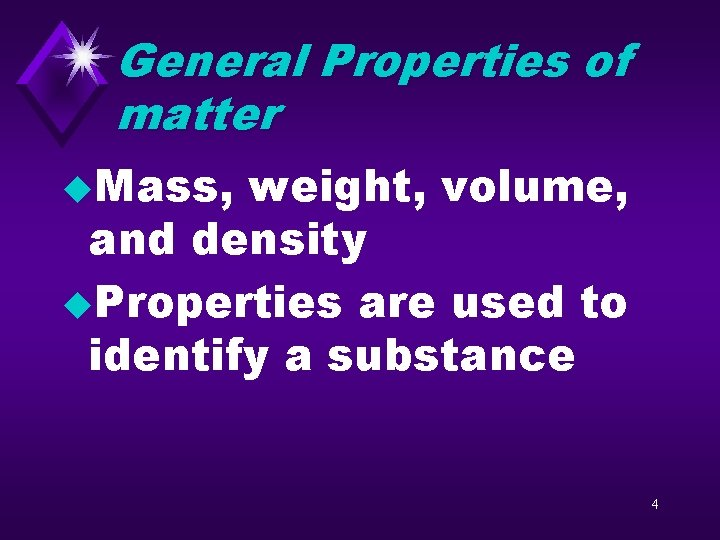General Properties of matter u. Mass, weight, volume, and density u. Properties are used to identify a substance 4What is mass? u. Mass is the amount of matter in an object u. Mass is constant u. Mass is also the measure of inertia 5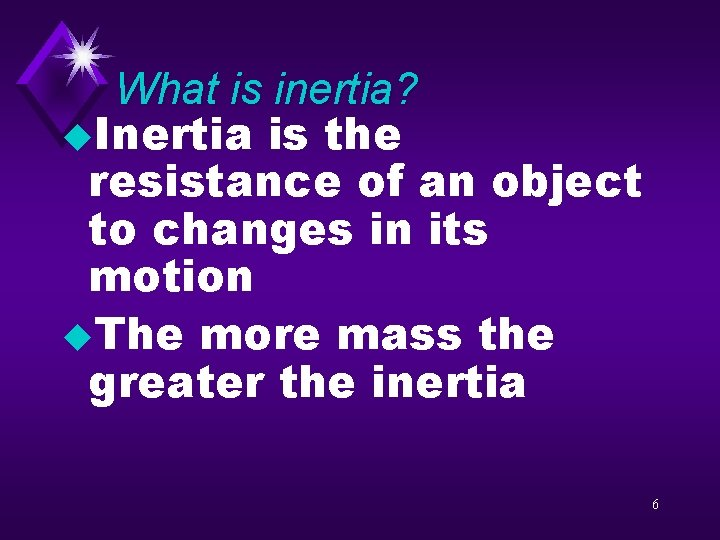What is inertia? u. Inertia is the resistance of an object to changes in its motion u. The more mass the greater the inertia 6Questions u. How is mass related to inertia? u. Why are properties of an object important? 7Question u. Which object has more inertia, an empty wagon or one loaded with rocks? Why? 9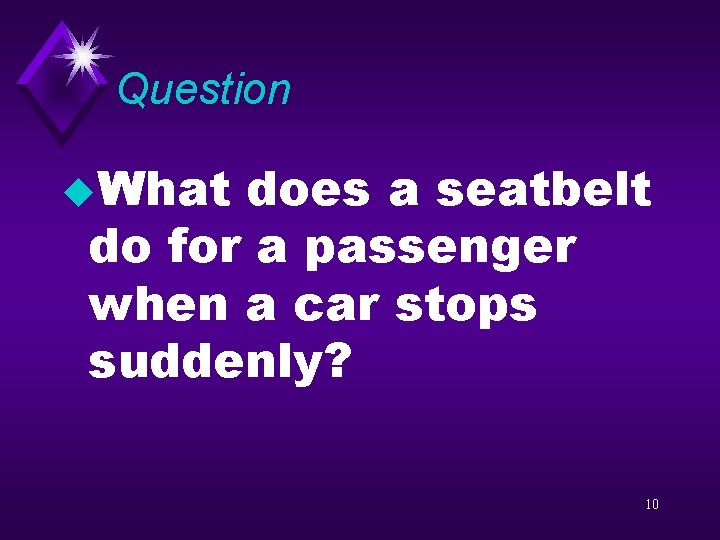Question u. What does a seatbelt do for a passenger when a car stops suddenly? 10Question u. Why would the passenger move forward without the restraining force of the belt? 11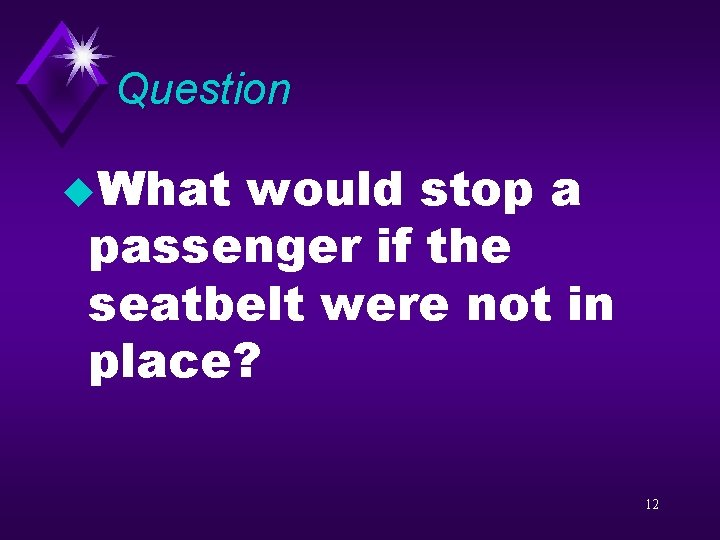Question u. What would stop a passenger if the seatbelt were not in place? 12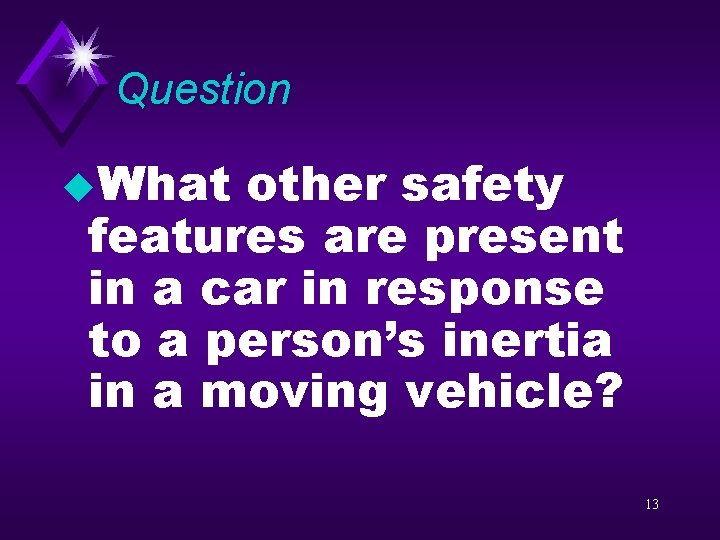Question u. What other safety features are present in a car in response to a person’s inertia in a moving vehicle? 13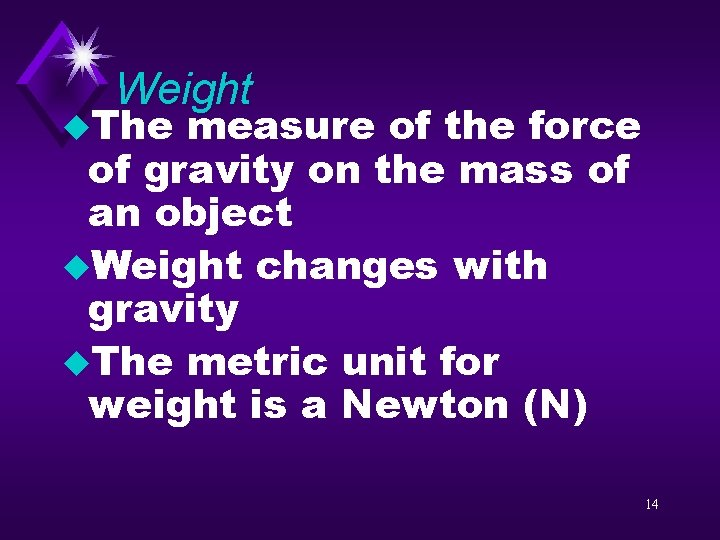Weight u. The measure of the force of gravity on the mass of an object u. Weight changes with gravity u. The metric unit for weight is a Newton (N) 14u 1 Weight formula kg = 2. 2 pounds u Weight is mass times gravity (9. 8 m/s 2) u W= m x g u What is your mass? u What is your weight in Newtons? 15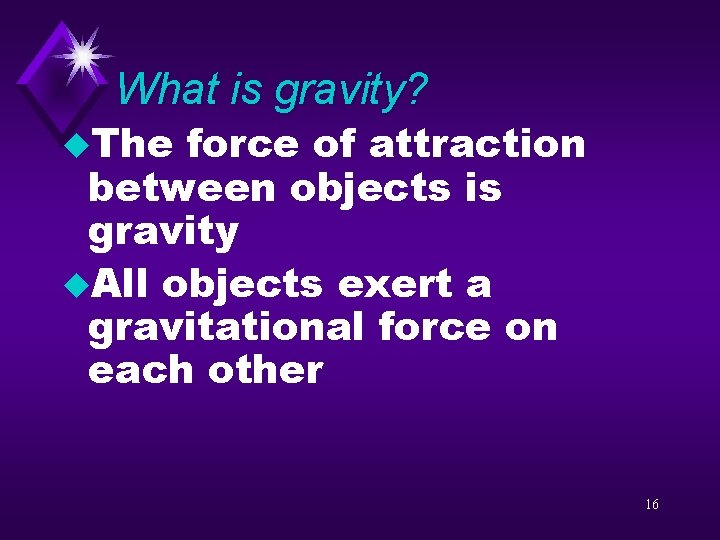What is gravity? u. The force of attraction between objects is gravity u. All objects exert a gravitational force on each other 16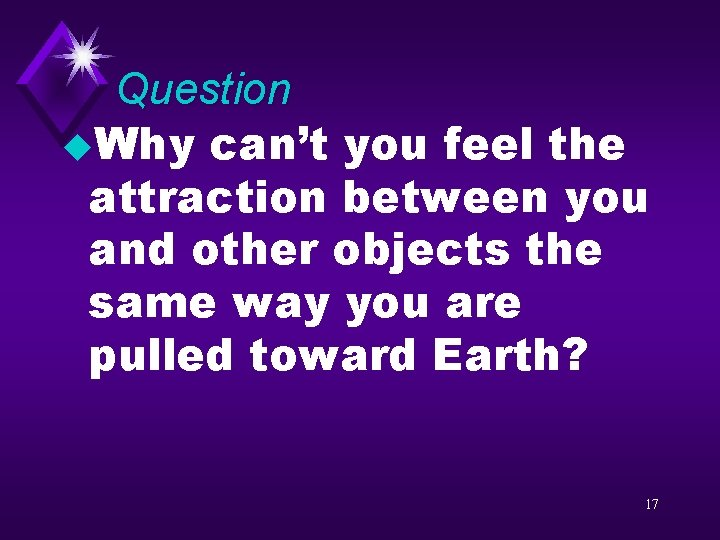Question u. Why can’t you feel the attraction between you and other objects the same way you are pulled toward Earth? 17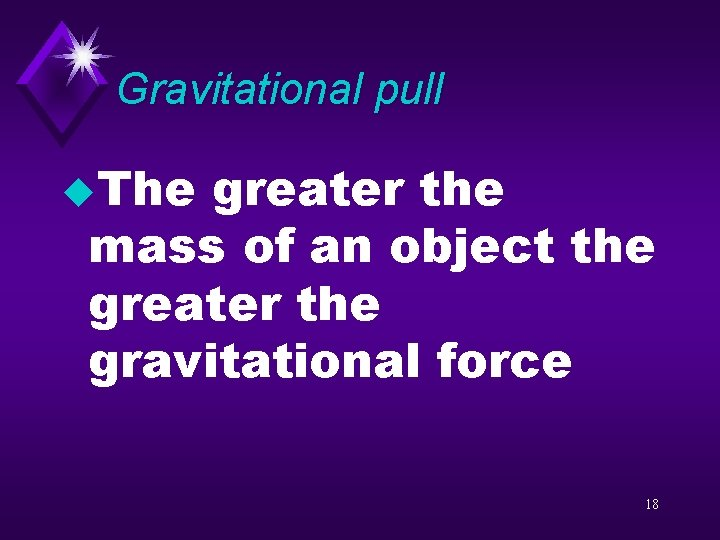Gravitational pull u. The greater the mass of an object the greater the gravitational force 18Question u. Why can’t we feel the pull of gravity from Jupiter even though it is so massive? 19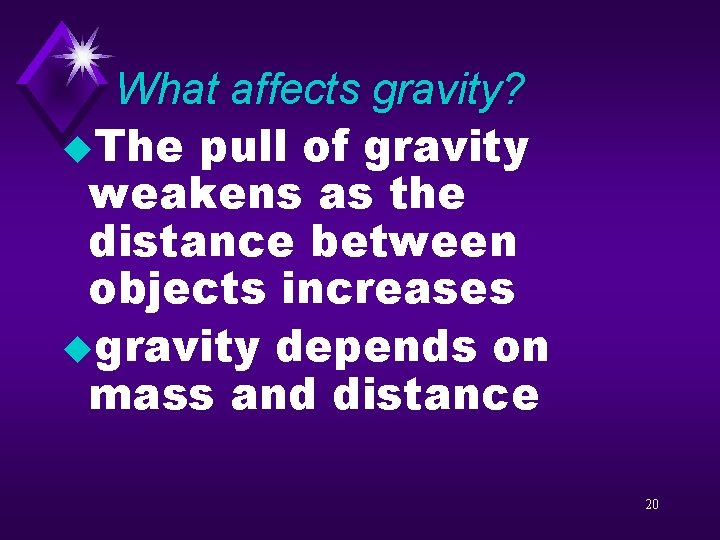What affects gravity? u. The pull of gravity weakens as the distance between objects increases ugravity depends on mass and distance 20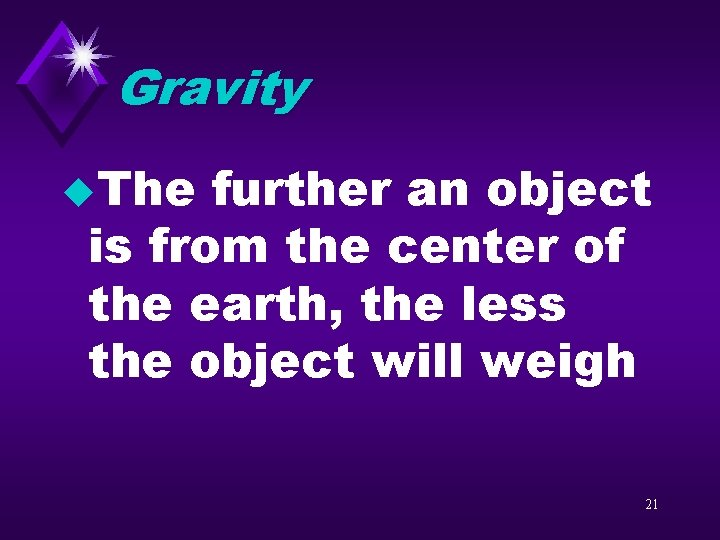Gravity u. The further an object is from the center of the earth, the less the object will weigh 21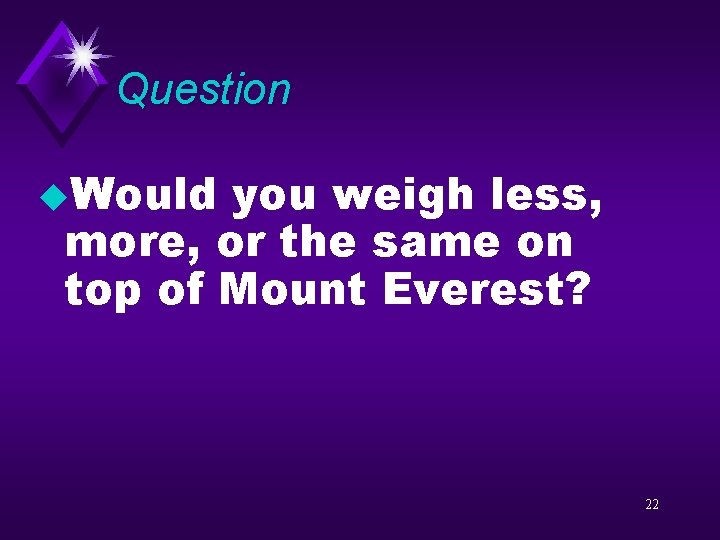Question u. Would you weigh less, more, or the same on top of Mount Everest? 22Question u. The moon is smaller than the earth. How would your weight be different on the moon? 23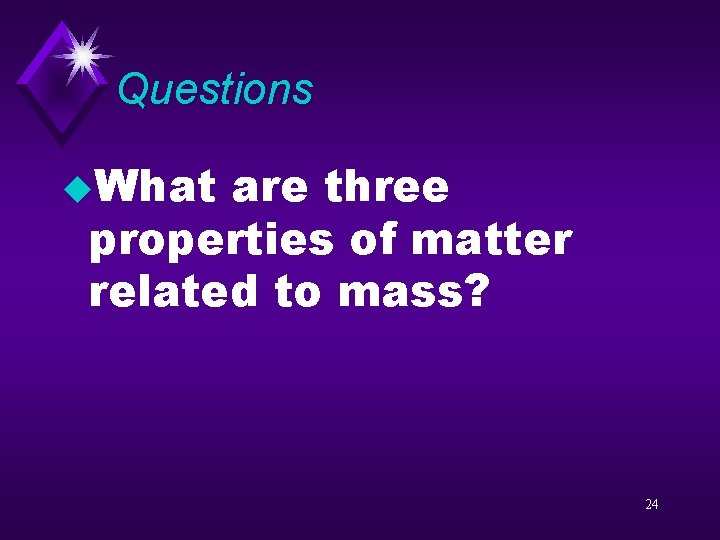Questions u. What are three properties of matter related to mass? 24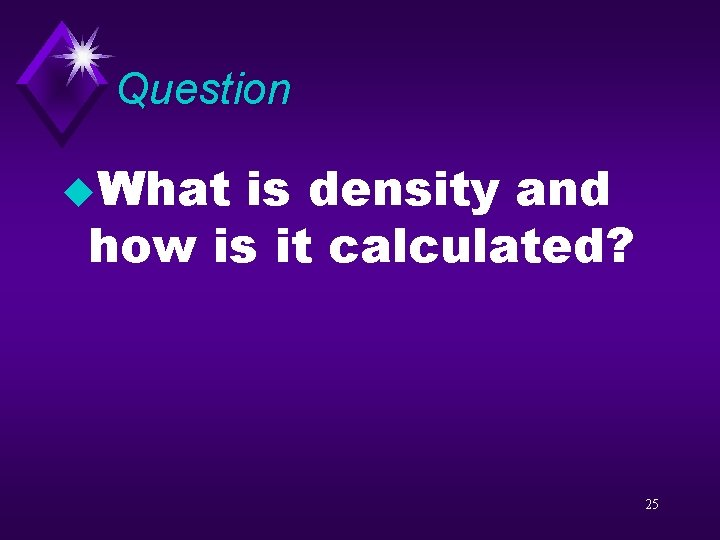Question u. What is density and how is it calculated? 25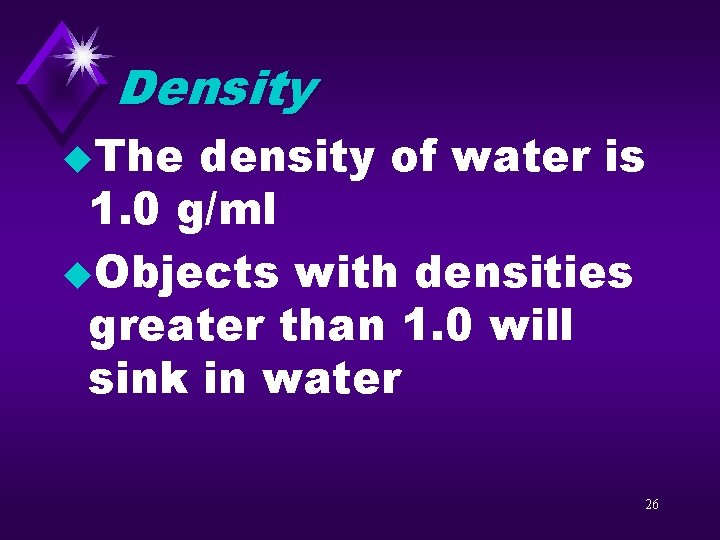Density u. The density of water is 1. 0 g/ml u. Objects with densities greater than 1. 0 will sink in water 26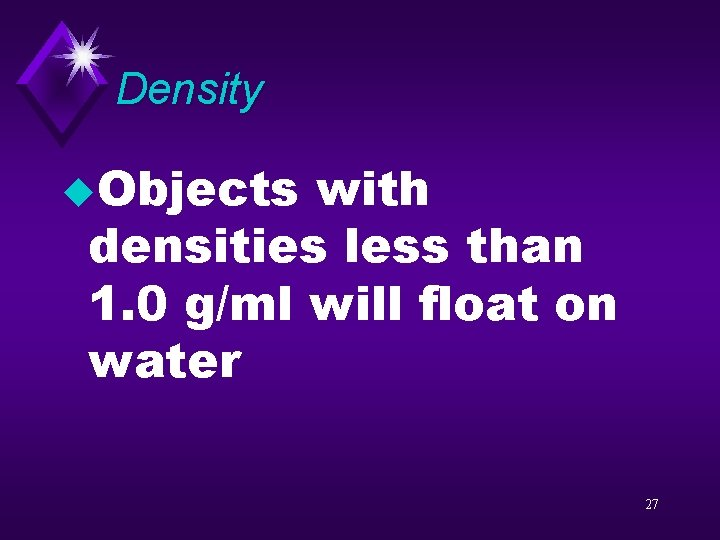Density u. Objects with densities less than 1. 0 g/ml will float on water 27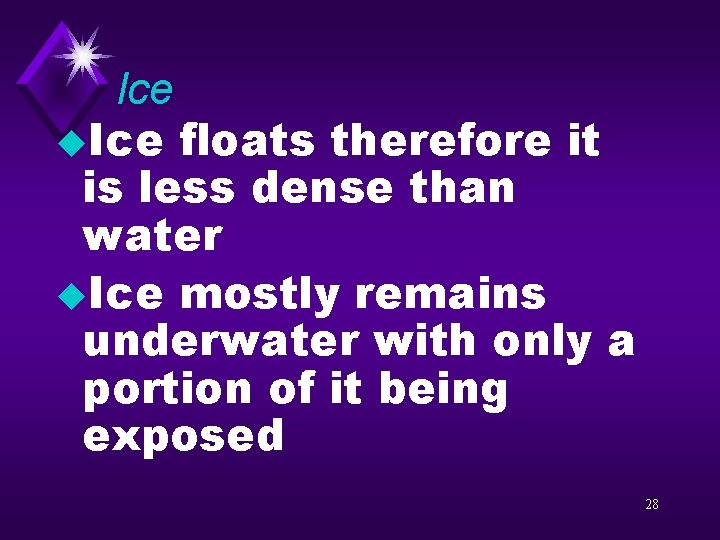Ice u. Ice floats therefore it is less dense than water u. Ice mostly remains underwater with only a portion of it being exposed 28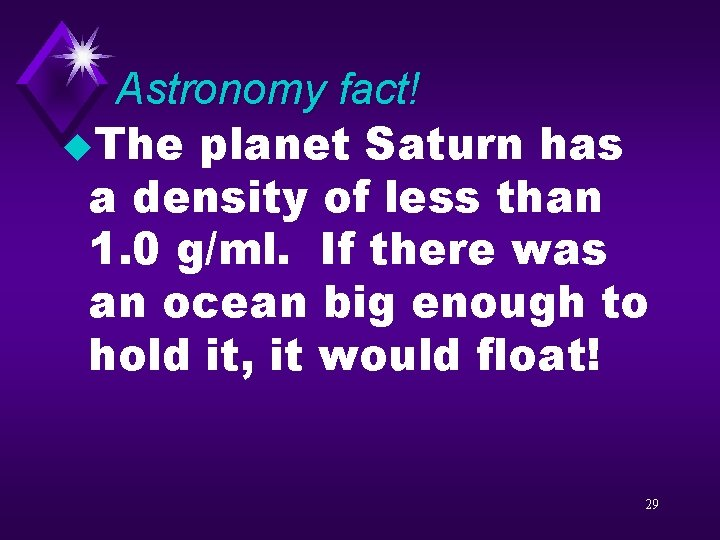Astronomy fact! u. The planet Saturn has a density of less than 1. 0 g/ml. If there was an ocean big enough to hold it, it would float! 29Calculations u. If 96. 5 grams of gold has a volume of 5 cubic centimeters, what is the density of gold? 30Calculation u. If 96. 5 g of aluminum has a volume of 35 cm 3, what is the density of aluminum? 31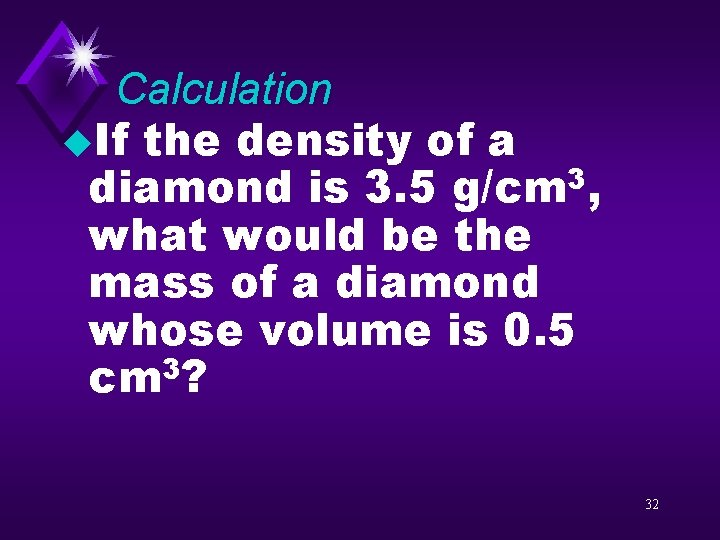Calculation u. If the density of a diamond is 3. 5 g/cm 3, what would be the mass of a diamond whose volume is 0. 5 3 cm ? 32Questions u. What is another name for a chemical change? u. Describe sublimation. u. How is a chemical change different from a physical change? 33